# CVPR2021|单个网络支持 sRGB 渲染与 RAW 数据重建，港科大陈启峰团队提出可逆 ISP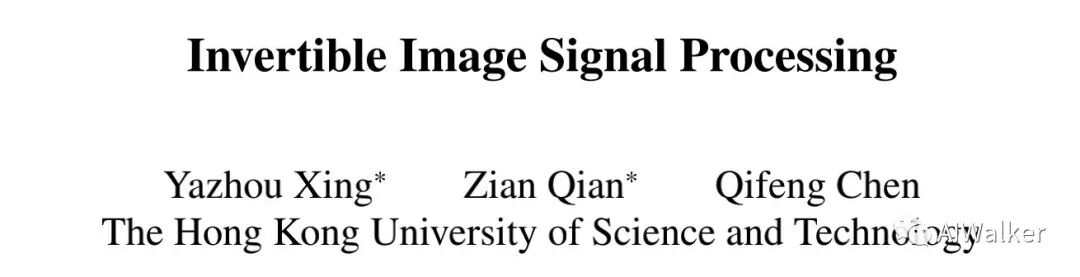## Abstract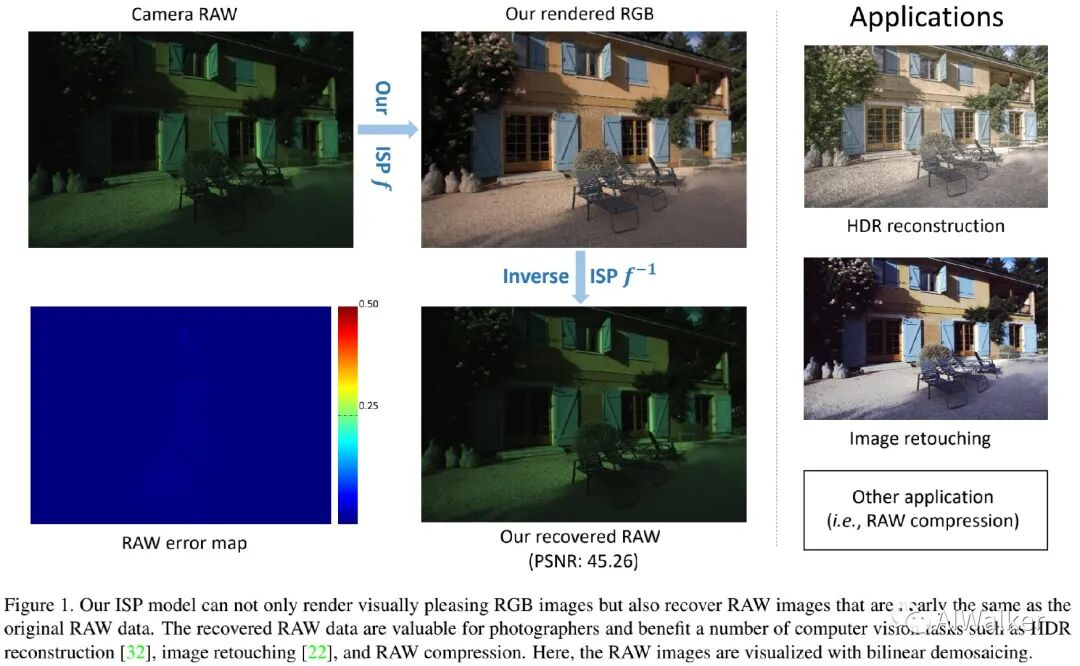• 首个从可逆ISP角度出发进行RAW数据重建的方案；
• 解决了ISP模块中的信息损失问题，且对JPEG压缩鲁棒；
• 在两个数码相机数据上验证了所提方案的有效性，并在多个应用场景(比如retouch、HDR等)验证了其潜在应用价值。

## Traditional ISP Analysis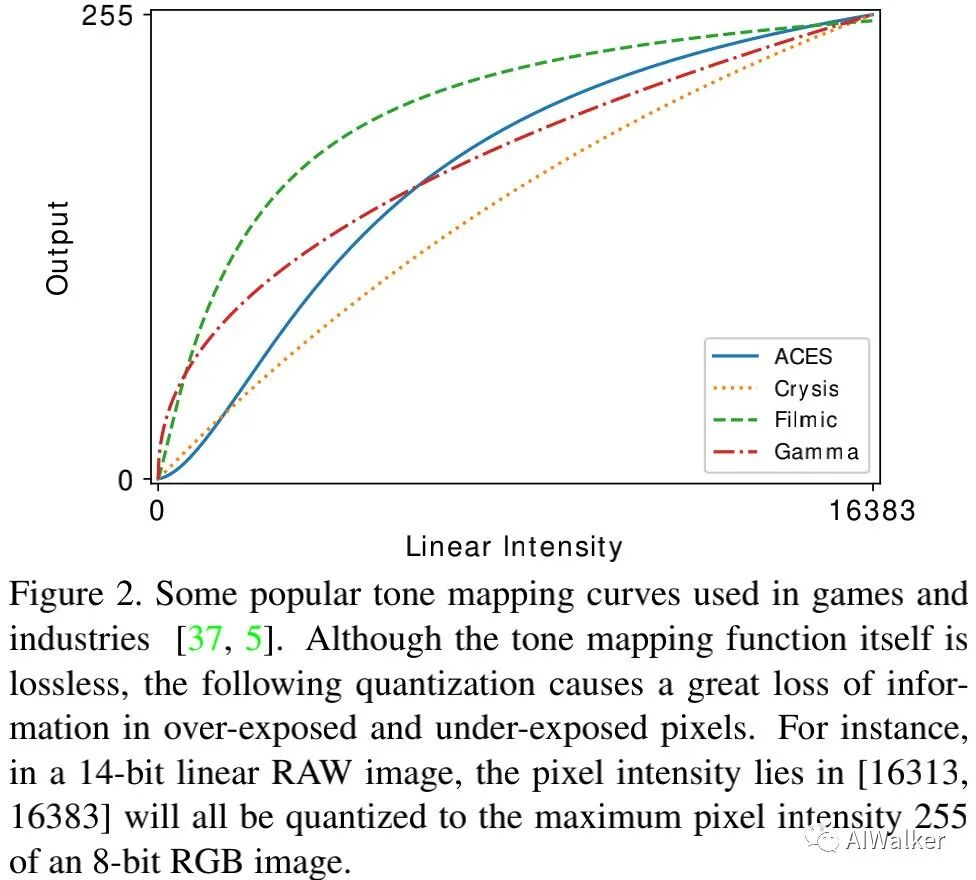• Quantization \& Tone Mapping。ISP的某些步骤(如去马赛克、gamma压缩)包含浮点操作，因此量化对于将数据转换到整数空间非常重要。比如，round操作理论上会带来的灰度误差。然而，tone mapping会进一步放大该灰度误差，上图给出了tonemapping曲线示意图。对于14-bit的raw数据，gamma压缩会使得范围的数据压缩到255灰度，该步骤会导致单像素0.004RMSE误差。因此，直接从8-bit的sRGB数据合成14-bit的RAW数据极具挑战；
• Out-range Value Clipping。数值截断是一种常用的将raw数值规范化到合理范围的操作，常见于色彩空间转换、去马赛克、降噪、tone mapping等操作。传统ISP需要独立的进行手动调节，导致了误差累积，进一步加剧了信息损失。
• JPEG Compression。现代数码相机往往将RGB图像保存为JPEG格式，这会进一步加剧RAW图像重建的难度。JPEG包含四个步骤：颜色空间转换、离散余弦变换、量化、熵编码。事实上，量化是JPEG仅有的存在损失且非可微分的步骤。由于JPEG信息损失难以逆转，我们采用了一种“妥协”方式：将JPEG压缩步骤集成到所提框架中以消除信息损失

## Invertible Image Signal Processing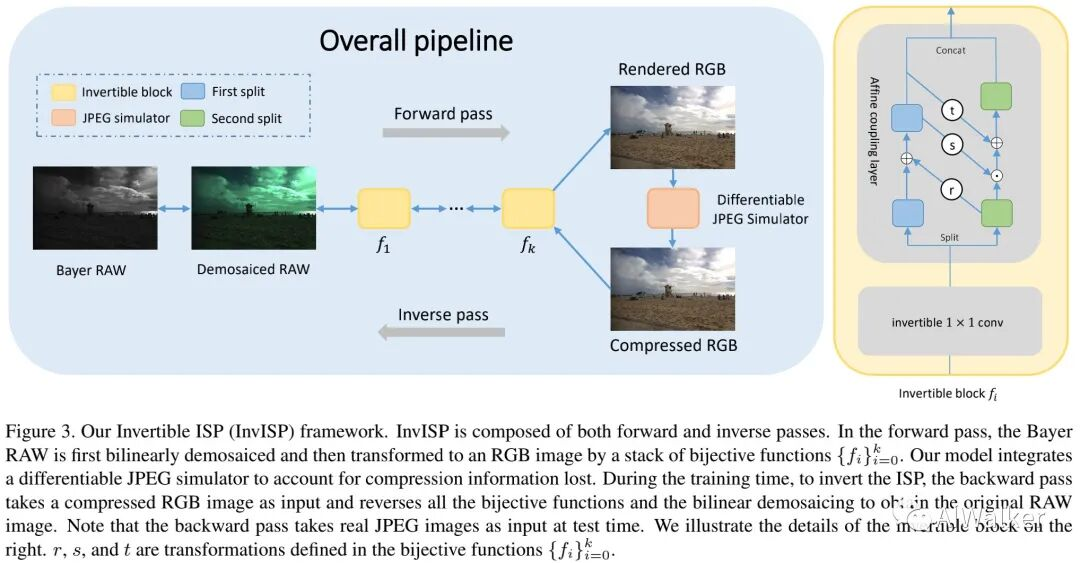$$y = f_0 \circ f_1 \circ f_2 \circ \cdots \circ f_k(x) \ x = fk^{-1} \circ f{k-1}^{-1} \circ \cdots \circ f_0^{-1}(y)$$

$$\mathbf{n}{1:d} = \mathbf{m}{1:d} \ \mathbf{n}{d+1:D} = \mathbf{m}{d+1:D} \odot exp(s(\mathbf{m}{1:d})) + t(\mathbf{m}{1:d})$$

$$\mathbf{m}{d+1:D} = (\mathbf{n}{d+1:D} - t(\mathbf{n}{1:d})) \odot exp(-s(\mathbf{n}{1:d})) \ \mathbf{m}{1:d} = \mathbf{n}{1:d} - r(\mathbf{m}_{d+1:D})$$

$$L = |f(x) - y|_1 + \lambda | f^{-1}(y) - x |_1$$

## Differentiable JPEG Simulator

$$Q(I) = I - \frac{1}{\pi} \sum_{k=1}^K \frac{(-1)^{k+1}}{k} sin(2\pi kI)$$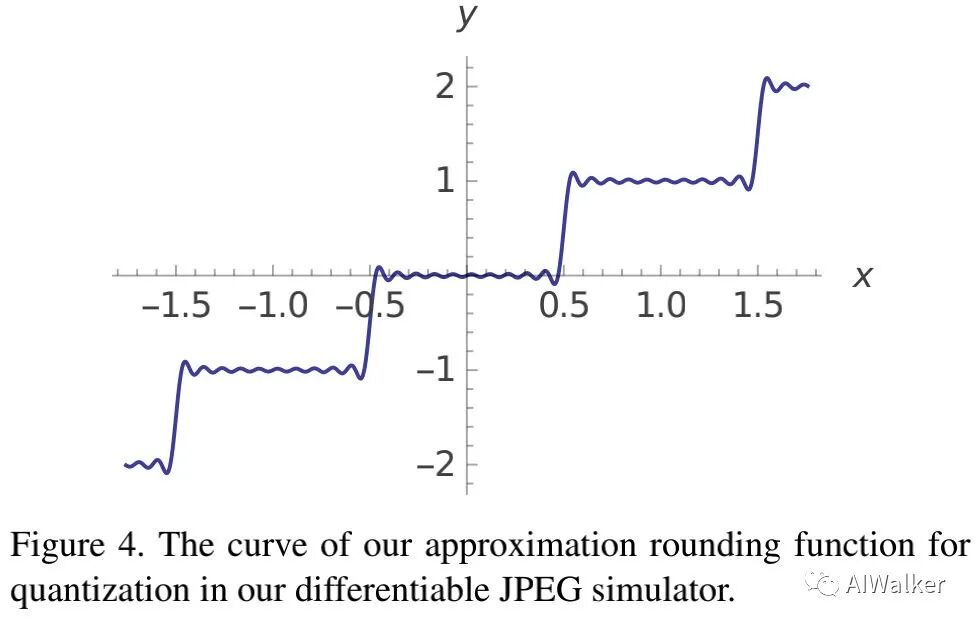## Experiments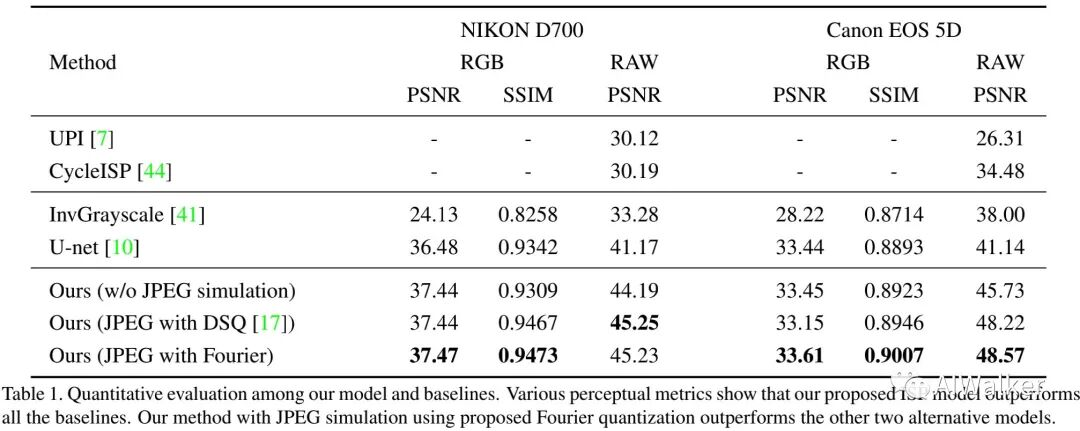• 相比UPI与CycleISP，所提方法可以重建更精确的RAW数据(PSNR甚至可以高出15dB)。这个结果并不惊讶：因为ISP中的信息损失是非常难以逆转的，这会导致比较差的合成Raw重加结果；而InvISP可以同时进行RGB与RAW重建优化。可以更好的处理量化、JPEG压缩以及数值截断等导致的信息损失。
• 相比InvGrayScale与UNet基线模型，所提方法同样具有更好的结果，这意味InvISP提供了一个更强的RAW数据重建方案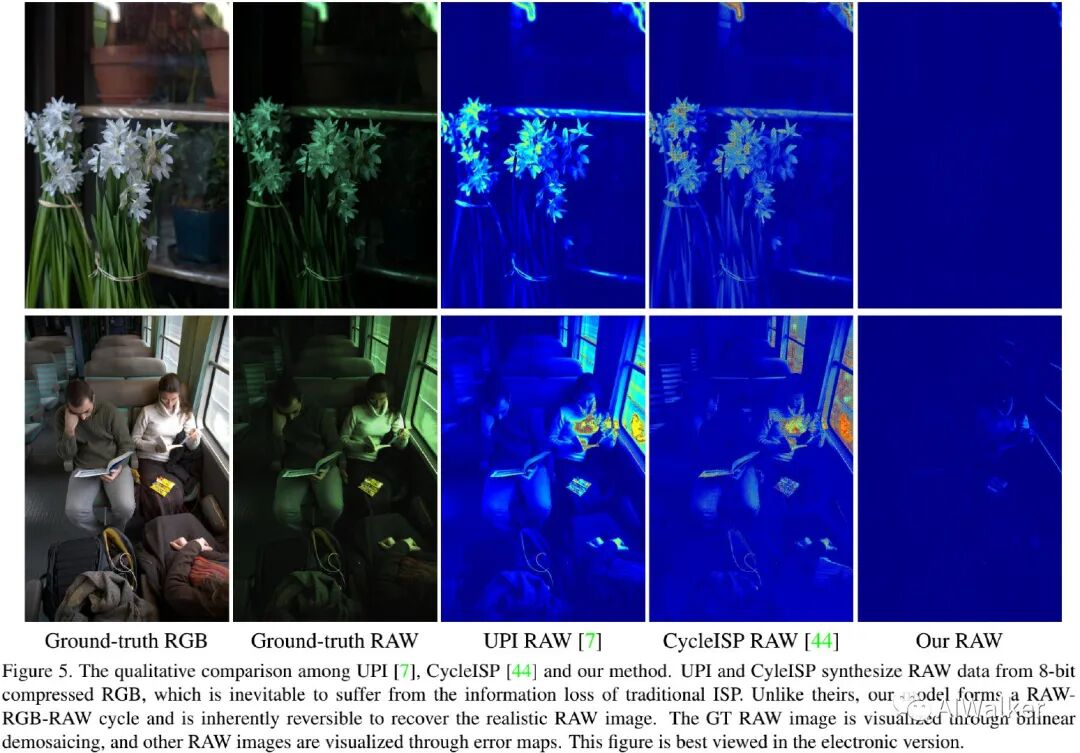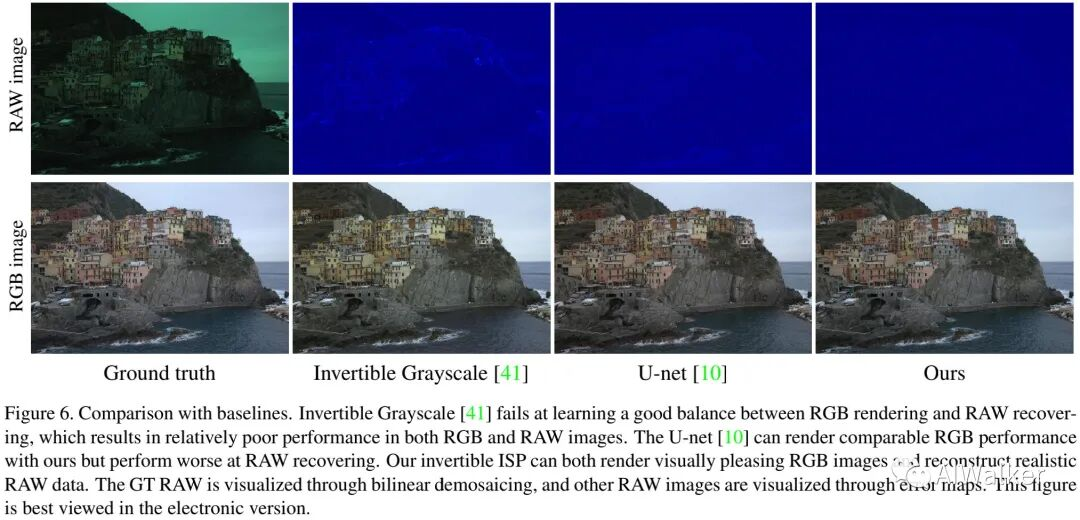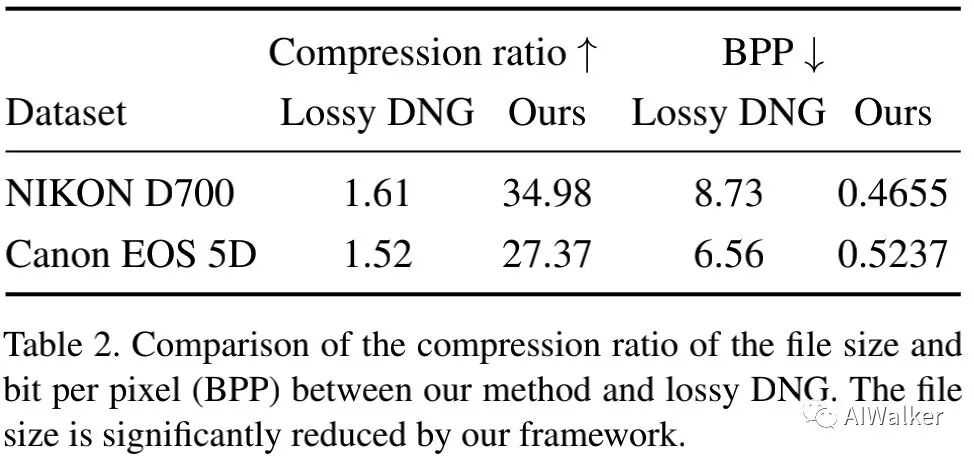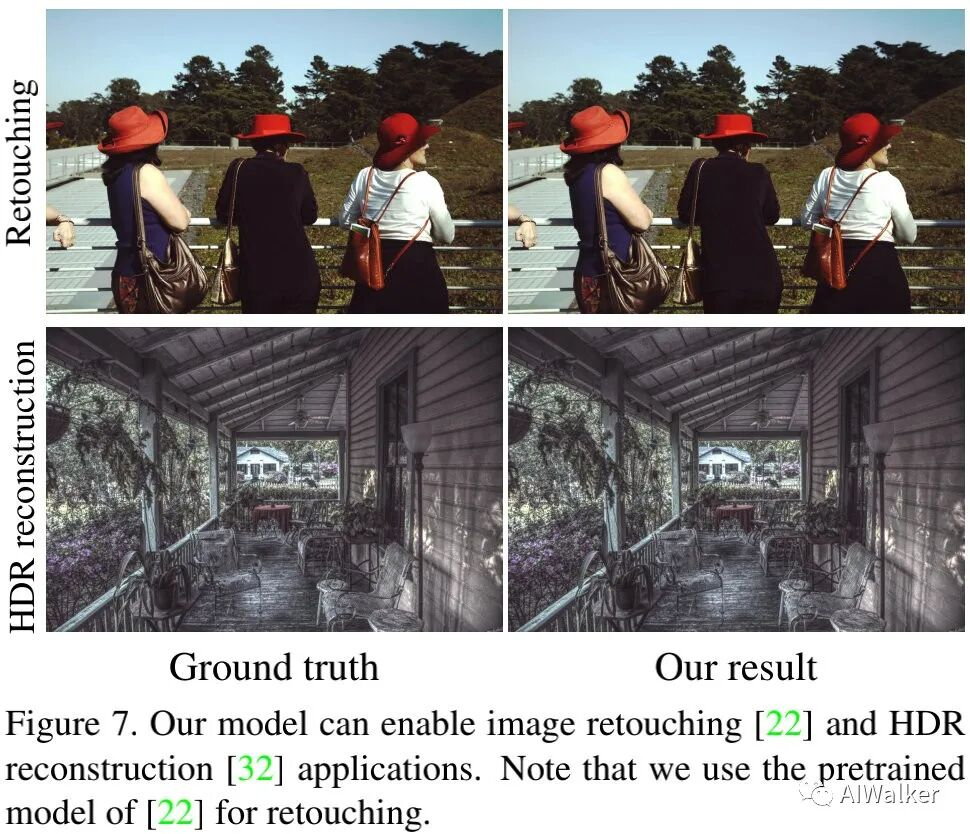• 重建RAW数据与相机拍摄的RAW具有无法区分的视觉质量；
• 所提方法可以消除HDR过程中的过曝区域\&欠曝区域的信息损失问题，进而取得更好的HDR重建结果。